# AP Chemistry : Identifying and Defining Acids and Bases

## Example Questions

← Previous 1

### Example Question #1 : Identifying And Defining Acids And Bases

Which of the following is a Lewis base?

Me3B

B2H6

NH3

BF3

NH3

Explanation:

NH3 should be the clear correct choice, since it is the only one having a pair of electrons that are available. The rest of the answer choices are all Lewis acids.

### Example Question #1 : Identifying And Defining Acids And Bases

What is the defining characteristic of Bronsted-Lowry bases?

Electron pair acceptor

Dissociates in solution to give the H+ ion

Proton (H+) donor

Proton (H+) acceptor

Dissociates in solution to give the OH– ion

Proton (H+) acceptor

Explanation:

The definition of a Bronsted-Lowry base is a species that has the ability to gain, or accept a proton (H+). Dissociating in solution is part of the Arrhenius definition of acids and bases, and Lewis acid are electron pair acceptors.

### Example Question #51 : Reaction Types

Which of the following can act as a Lewis base?

NH2NH2

CH3COOH

HF

BF3

NH2NH2

Explanation:

A Lewis base is an electron-pair donor. N atoms have a valence of 5, and in the NH2NH2 compound, it is only bonded to another N atom and 2 H, so it's only using 3 of its 5 valence electrons to form these bonds. Thus, each nitrogen has a pair of unbonded electrons and can act as a Lewis base.

### Example Question #2 : Identifying And Defining Acids And Bases

In the following equation, which is the conjugate base of HClO4?

HClO4 + H2O → ClO4– + H3O+

H3O+

H2O

none of the above

ClO4–

HClO4

ClO4–

Explanation:

The conjugate base of an acid will be the same compound, short one H atom. ClO4– is the only one that meets this criterion.

### Example Question #45 : Acid Base Reactions

Which of the following is a Lewis base?

CH3CH2CH2CH3

PH3

NH4+

CH2COOH

PH3

Explanation:

A Lewis base is an electron-pair donor. Only PH3 has a pair of nonbonding electrons and can act as a donor.

### Example Question #1 : Identifying And Defining Acids And Bases

Which of the following is the conjugate base of oxalic acid (H2C2O4)?

HC2O4+

HC2O4–

(C2O4) 2–

H2C2O4

HC2O4–

Explanation:

The conjugate base has one less H atoms and one unit greater negative charge because of this. Thus, the correct answer is HC2O4–

### Example Question #52 : Reaction Types

Which of the following solutions will have a pH greater than 7?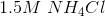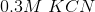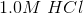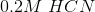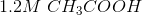Explanation:

HCN, HCl, CH3COOH, and NH4Cl are all acids (NH4+ is the ammonium ion). That only leaves KCN as the correct answer.

### Example Question #1 : Identifying And Defining Acids And Bases

BCl3 is a                     .

Bronsted-Lowry acid

Lewis base

Arrhenius acid

Bronsted-Lowry base

Lewis acid

Lewis acid

Explanation:

A Lewis acid is a species that can accept an electron pair. In the BCl3 molecule, B does not have a complete octet (3 covalent bonds, thus 6 electrons around it rather than 8). Thus, it can accept another electron pair, making it a Lewis acid.

### Example Question #51 : Acid Base Reactions

Which of the following compounds is a Lewis acid:,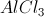,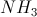,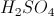?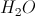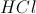Explanation:

A Lewis acid is a 2-electron acceptor, and a Lewis base is a 2-electron donor., while it is acidic, cannot accept two electrons, so it is not a Lewis acid.can accept two electrons, so it is a Lewis acid.is a Lewis base., like hydrochloric acid, is acidic, but cannot accept two electrons, so it is not a Lewis acid.

### Example Question #55 : Acid Base Reactions

Which of the following salts will result in an acidic solution?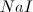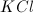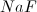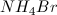Explanation:

All of the listed salts will dissolve into ions when in water. When the ions are in solution, they can act as acids or bases by donating or accepting protons. Chloride, bromide, and iodide ions are all conjugate bases of strong acids, so they will not accept protons. Sodium and potassium ions are the conjugate acids of strong bases, which dissociate completely, so they will not accept hydroxide ions.

Ammonium is the conjugate acid of ammonia, a weak base. The ammonium ion can donate a proton to the solution. This will make the solution slightly acidic. As a result, ammonium bromide is a salt that will make an acidic solution.

← Previous 1

### All AP Chemistry Resources